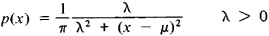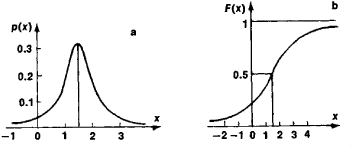# Cauchy Distribution

(redirected from Lorentzian distribution)

## Cauchy distribution

[kō·shē dis·trə′byü·shən]
(statistics)
A distribution function having the form M /[π M 2+ (x-a)2], where x is the variable and M and a are constants. Also known as Cauchy frequency distribution.

## Cauchy Distribution

a special type of probability distribution of random variables. Introduced by Cauchy, it is marked by the densityThe characteristic function is

f(t) = exp (μit − λ ǀ t ǀ)

The Cauchy distribution is unimodal and symmetric with respect to the point x = μ, which is its mode and median. NoFigure 1. Cauchy distribution: (a) probability density, (b) distribution function

moments of positive order of a Cauchy distribution exist. Figure 1 depicts a Cauchy distribution for μ = 1.5 and λ = 1.

Mentioned in ?
References in periodicals archive ?
Considering the Lorentzian distribution, the energy density of particle-like static spherically symmetric object with mass M has the following form :
This paper investigates noncommutative wormhole solutions with Lorentzian distribution in F(T, [T.sub.G]) gravity.
Bhar and Rahaman  examined in GR whether the wormhole solutions exist in different dimensional non-commutative space-time with Lorentzian distribution. They found that wormhole solutions appear only for four and five dimensions but no solution exists for higher dimensions.
When Doppler (Gaussian) component is constant and small, Lorentzian distribution will prevail.
Lorentzian distribution function was applied for resolution of the bands, according to Equation 1.
The curves were deconvoluted without a base line by the method of least squares curve-fitting using Lorentzian distribution function, according to Equation 2.
Thus, depending on the resolution of the spectrometer, there is no evidence that the line shape function satisfies a purely Gaussian or a purely Lorentzian distribution function.
An observed x-ray peak will be described as the sum of many pseudo-Voigt profiles, each of them being characterized by four parameters, the proportion Cg of Gaussian to Lorentzian distribution, the peak position, the peak width and the peak amplitude.
The proportion of Gaussian and Lorentzian distributions in the peak profile is a function of the intrinsic properties of the analyzed emission, i.e., of the energy sub-shells involved in the analyzed radiative transitions.

Site: Follow: Share:
Open / Close